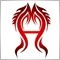# How to get an int value from Indicator?1141

When we want to get value from an Indicator, we always should use iCustom() to get, and the value must be stored in a double array which also should be defined as indicator buffer; so when we can only get double value from Indicator by iCustom(), how about to get an int value from an Indicator?

maybe we can save the int value in a double array in Indicator and call iCustom() to get by int variable in EA, that is OK?

in the addition, I just made an Indicator which can give the trade signal according to some trade logic, and then the signal value can be received in EA; in the fact the signal is 1 or -1; if -1, EA will sell, and 1, EA will buy. I tested this EA and get a result report; after that, I made another EA which trade according to the same logic of first one(in the fact, some key code is just copied from the first one); and I have checked very carefully, and confirm that these two EA have the same logic; what is amazing is that their reports is very different; I find that there are many chance in which trades should be done but in the fact not in the first EA. I don';t know why, I suspect there is something wrong in the first EA because of indicator called in EA25310

vx0532: maybe we can save the int value in a double array in Indicator and call iCustom() to get by int variable in EA, that is OK?

Of course it is.

ArrayCopyRates stores datetime (time,) doubles (OHLC) and int (volume) in the same double array.1141

WHRoeder:

Of course it is.

ArrayCopyRates stores datetime (time,) doubles (OHLC) and int (volume) in the same double array.

Thanks.

In the addition, for the same trade logic, one case I code in EA and test it get result A; another case I code in Indicator, and call the indicator by iCustom() to trade, and then test it, get result B;

A is very different from B, orderstotal in A is 40, but in B is 21; what is the most possibility for this difference?2397

That is really not possible to say without seeing and testing your code.1141

SDC:

That is really not possible to say without seeing and testing your code.

EA (not call iCustom()) is as below:

```extern int Seek_Period=15,Mini_Swing=60,Trade_Range=90;
int Stop_Loss=80;
double Get_High;

int init()
{
return(0);
}
int deinit()
{
return(0);
}

int start()
{
Get_High_Low();
if(Get_High!=0&&OrdersTotal()==0)
OrderSend(Symbol(),OP_SELL,1,Bid,3,Bid+Stop_Loss*Point,Bid-3*Stop_Loss*Point,0,0,0,0);
return(0);
}

void Get_High_Low()
{
int P_CNT=Seek_Period;
Get_High=0.0;
int G_i=0;
while(P_CNT>=6)
{
G_i=iHighest(NULL,0,MODE_HIGH,P_CNT,0);
double High_Refer_P=High[iHighest(NULL,0,MODE_HIGH,10,G_i-5)],Low_Refer_P=Low[iLowest(NULL,0,MODE_LOW,G_i,0)];
double HL_Current_P=High[iHighest(NULL,0,MODE_HIGH,3,0)];
if(
G_i>=5&&MathAbs(High_Refer_P-High[G_i])<Point&&High[G_i]-Low_Refer_P>=Mini_Swing*Point&&HL_Current_P!=High
)  {Get_High=High[G_i];break;}

P_CNT--;
}
return;
}
```

EA (call Indicator by iCustom) all codes are below(part I EA codes and part II Indicator codes):

part I

```int Stop_Loss=80;
double Get_High;

int init()
{
return(0);
}

int deinit()
{
return(0);
}

int start()
{
Get_High=iCustom(NULL,0,"Test_Ind",15,60,90,1,0);
if(Get_High!=0&&OrdersTotal()==0)
OrderSend(Symbol(),OP_SELL,1,Bid,3,Bid+Stop_Loss*Point,Bid-3*Stop_Loss*Point,0,0,0,0);
return(0);
}
```

part II

```#property indicator_chart_window
#property indicator_buffers 1
#property indicator_color1 Yellow

double High_Point[];
double Call;

int init()
{
IndicatorBuffers(2);
SetIndexBuffer(0,High_Point);
SetIndexStyle(0,DRAW_SECTION);
SetIndexEmptyValue(0,0.0);
SetIndexBuffer(1,Call);
return(0);
}
int deinit()
{
return(0);
}

int start()
{
if(Bars<=Seek_Period) return(0);
int Counted_Bars=IndicatorCounted();
if(Counted_Bars==0) Counted_Bars--;
int Limit=Bars-Counted_Bars;
ArrayInitialize(Call,0.0);
for(int i=Limit-1;i>0;i--)
{
int P_CNT=Seek_Period;
int G_i=0;
while(P_CNT>=6)
{
G_i=iHighest(NULL,0,MODE_HIGH,P_CNT,0);
double High_Refer_P=High[iHighest(NULL,0,MODE_HIGH,10,G_i-5)],Low_Refer_P=Low[iLowest(NULL,0,MODE_LOW,G_i,0)];
double HL_Current_P=High[iHighest(NULL,0,MODE_HIGH,3,0)];
if(
G_i>=5&&MathAbs(High_Refer_P-High[G_i])<Point&&High[G_i]-Low_Refer_P>=Mini_Swing*Point&&HL_Current_P!=High
)  {Call=High[G_i];break;}
P_CNT--;
}
}

return(0);
}
```

what i want to explain is that: the two EA 's logic' are same, and most codes are same, just copy and paste and I also mark the exactly same codes in the two EA red to save your time to compare the two EA.

but the result is very different; I test them on USDJPY from 2013.11.20 to 2013.12.4 on M5 chart, the first one trades 67 orders and the second one trades 15 orders.

I can't understand this situation. how do you think the difference?17400

vx0532:

EA (not call iCustom()) is as below:

EA (call Indicator by iCustom) all codes are below(part I EA codes and part II Indicator codes):

part I

part II

what i want to explain is that: the two EA 's logic' are same, and most codes are same, just copy and paste and I also mark the exactly same codes in the two EA red to save your time to compare the two EA.

but the result is very different; I test them on USDJPY from 2013.11.20 to 2013.12.4 on M5 chart, the first one trades 67 orders and the second one trades 15 orders.

I can't understand this situation. how do you think the difference?

What is the value of buffer 1, Call, if you don't assign it a value ?

```int init()
{
IndicatorBuffers(2);

SetIndexBuffer(0,High_Point);
SetIndexStyle(0,DRAW_SECTION);

SetIndexEmptyValue(0,0.0);

SetIndexBuffer(1,Call);
return(0);```1141

RaptorUK:

What is the value of buffer 1, Call, if you don't assign it a value ?

I have initialized it; so it should be 0.0 if not assign it a value.
`ArrayInitialize(Call,0.0);`17400

vx0532:
I have initialized it; so it should be 0.0 if not assign it a value.
The code I posted is from the Indicator . . . and I said buffer not array.1141

RaptorUK:
The code I posted is from the Indicator . . . and I said buffer not array.

you mean arrayinitialize() can never be used in Indicator?

array in Indicator must be assigned if we want to use it,yes>?17400

vx0532:

you mean arrayinitialize() can never be used in Indicator?

array in Indicator must be assigned if we want to use it,yes>?

What I mean is . . .

RaptorUK:

What is the value of buffer 1, Call, if you don't assign it a value ?

Do you know the answer to my question ?1141

RaptorUK:

What I mean is . . .

Do you know the answer to my question ?

sorry, I don't know your question exactly, could you told me how shall I modify my codes?

if I don't assign it a value, I think Call is 0.0? yes?